# 6. Synthetic Long & Arbitrage

## 6.1 – Background

Imagine a situation where you would be required to simultaneously establish a long and short position on Nifty Futures, expiring in the same series. How would you do this and more importantly why would you do this?

We will address both these questions in this chapter. To begin with let us understand how this can be done and later move ahead to understand why one would want to do this (if you are curious, arbitrage is the obvious answer).

Options as you may have realized by now, are highly versatile derivative instruments; you can use these instruments to create any kind of payoff structure including that of the futures (both long and short futures payoff).

In this chapter we will understand how we can artificially replicate a long futures pay off using options. However before we proceed, you may want to just review the long Future’s ‘linear’ payoff here

Alternatively, here is a quick overview –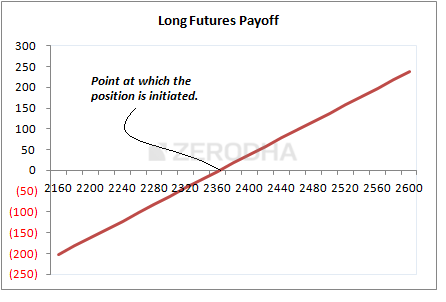As you can see, the long futures position has been initiated at 2360, and at that point you neither make money nor lose money, hence the point at which you initiate the position becomes the breakeven point. You make a profit as the futures move higher than the breakeven point and you make a loss the lower the futures move below the breakeven point. The amount of profit you make for a 10 point up move is exactly the same as the amount of loss you’d make for a 10 point down move. Because of this linearity in payoff, the future is also called a linear instrument.

The idea with a Synthetic Long is to build a similar long Future’s payoff using options.

## 6.2 – Strategy Notes

Executing a Synthetic Long is fairly simple; all that one has to do is –

1. Buy the ATM Call Option
2. Sell the ATM Put Option

When you do this, you need to make sure –

1. The options belong to the same underlying
2. Belongs to the same expiry

Let us take an example to understand this better. Assume Nifty is at 7389, which would make 7400 the ATM strike. Synthetic Long would require us to go long on 7400 CE, the premium for this is Rs.107 and we would short the 7400 PE at 80.

The net cash outflow would be the difference between the two premiums i.e 107 – 80 = 27.

Let us consider a few market expiry scenarios –

Scenario 1 – Market expires at 7200 (below ATM)

At 7200, the 7400 CE would expire worthless, hence we would lose the premium paid i.e Rs.107/-. However the 7400 PE would have an intrinsic value, which can be calculated as follows –

Intrinsic value of Put Option = Max [Strike-Spot, 0]

= Max [7400 – 7200, 0]

=Max [200, 0]

= 200.

Clearly, since we are short on this option, we would lose money from the premium we have received. The loss would be –

80 – 200 = -120

Total payoff from the long Call and short Put position would be –

= -107 – 120

= -227

Scenario 2 – Market expires at 7400 (At ATM)

If the market expires exactly at 7400, both the options would expire worthless and hence –

1. We lose the premium paid for the 7400 CE option i.e 107
2. We get the retain the premium for the 7400 PE option i.e 80
3. Net payoff from both the positions would be -27e 80 – 107

Do note, 27 also happens to be the net cash outflow of the strategy, which is also the difference between the two premiums

Scenario 3 – Market expires at 7427 (ATM + Difference between the two premiums)

7427 is an interesting level, this is the breakeven point for the strategy, where we neither make money nor lose money.

1. 7400 CE – the option is ITM and has an intrinsic value of 27. However we have paid 107 as premium hence we experience a total loss of 80
2. 7400 PE – the option would expire OTM, hence we get to retain the entire premium of 80.
3. On one hand we make 80 and the other we lose 80. Hence we neither make nor lose any money, making 7427 the breakeven point for this strategy.

Scenario 4 – Market expires at 7600 (above ATM)

At 7600, the 7400 CE would have an intrinsic value of 200, we would make –

= 200 – 107

= 93

The 7400 PE would expire worthless; hence we get to retain the entire premium of Rs.80.

Total payoff from the strategy would be –

= 93 + 80

= 173

With the above 4 scenarios, we can conclude that the strategy makes money while the market moves higher and loses money while the market goes lower, similar to futures. However this still does not necessarily mean that the payoff is similar to that of futures. To establish that the synthetic long payoff behaves similar to futures, we need evaluate the payoff of the strategy with reference to the breakeven point; let’s say 200 point above and below the breakeven point. If the payoff is identical, then clearly there is linearity in the payoff, similar to futures.

So let’s figure this out.

We know the breakeven point for this is –

ATM + difference between the premiums

= 7400 + 27

= 7427

The payoff around this point should be symmetric. We will consider 7427 + 200 = 7627 and 7427-200 = 7227 for this.

At 7627 –

1. The 7400 CE would have an intrinsic value of 227, hence we get to make 227 – 107 = 120
2. The 7400 PE would expire worthless, hence we get to keep the entire premium of 80
3. In all we experience a payoff of 120 + 80 = 200

At 7227 –

1. The 7400 CE would not have any intrinsic value, hence we lose the entire premium paid i.e 107
2. The 7400 PE would have an intrinsic value of 7400 – 7227 = 173, since we have received 80 as premium the net loss would be 80 – 173 = -93.
3. In all we experience a payoff of -93-107 = -200

Clearly, there is payoff symmetry around the breakeven, and for this reason, the Synthetic Long mimics the payoff of the long futures instrument.

Further, here is the payoff at various expiry levels –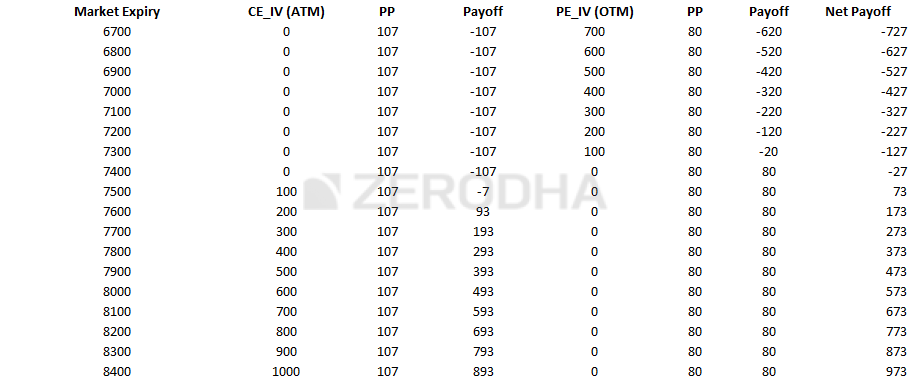And when you plot the Net Payoff, we get the payoff structure which is similar to the long call futures.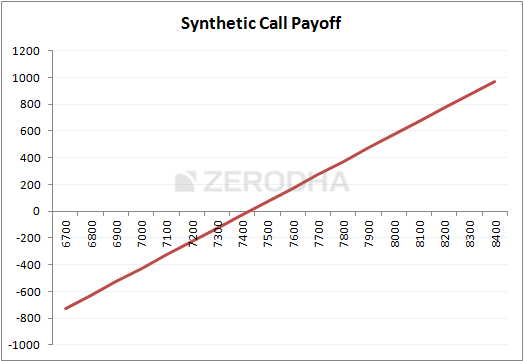Having figured out how to set up a Synthetic long, we need to figure out the typical circumstances under which setting up a synthetic long is required.

## 6.3 – The Fish market Arbitrage

I’ll assume that you have a basic understanding on Arbitrage. In easy words, arbitrage is an opportunity to buy goods/asset in a cheaper market and sell the same in expensive markets and pocket the difference in prices. If executed well, arbitrage trades are almost risk free. Let me attempt to give you a simple example of an arbitrage opportunity.

Assume you live by a coastal city with abundant supply of fresh sea fish, hence the rate at which fish is sold in your city is very low, let’s say Rs.100 per Kg. The neighboring city which is 125 kms away has a huge demand for the same fresh sea fish. However, in this neighboring city the same fish is sold at Rs.150 per Kg.

Given this if you can manage to buy the fish from your city at Rs.100 and manage to sell the same in the neighboring city at Rs.150, then in process you clearly get to pocket the price differential i.e Rs.50. Maybe you will have to account for transportation and other logistics, and instead of Rs.50, you get to keep Rs.30/- per Kg. This is still a beautiful deal and this is a typical arbitrage in the fish market!It looks perfect, think about it – if you can do this everyday i.e buy fish from your city at Rs.100 and sell in the neighboring city at Rs.150, adjust Rs.20 towards expenses then Rs.30 per KG is guaranteed risk free profit.

This is indeed risk free, provides nothing changes. But if things change, so will your profitability, let me list few things that could change –

1. No Fish (opportunity risk) – Assume one day you go to the market to buy fish at Rs.100, and you realize there is no fish in the market. Then you have no opportunity to make Rs.30/-.
2. No Buyers (liquidity risk) – You buy the fish at Rs.100 and go to the neighboring town to sell the same at Rs.150, but you realize that there are no buyers. You are left holding a bag full of dead fish, literally worthless!
3. Bad bargaining (execution risk) – The entire arbitrage opportunity hinges upon the fact that you can ‘always’ bargain to buy at Rs.100 and sell at Rs.150. What if on a bad day you happen to buy at 110 and sell at 140? You still have to pay 20 for transport, this means instead of the regular 30 Rupees profit you get to make only 10 Rupees, and if this continues, then the arbitrage opportunity would become less attractive and you may not want to do this at all.
4. Transport becomes expensive (cost of transaction) – This is another crucial factor for the profitability of the arbitrage trade. Imagine if the cost of transportation increases from Rs.20 to Rs.30? Clearly the arbitrage opportunity starts looking less attractive as the cost of execution goes higher and higher. Cost of transaction is a critical factor that makes or breaks an arbitrage opportunity
5. Competition kicks in (who can drop lower?) – Given that the world is inherently competitive you are likely to attract some competition who would also like to make that risk free Rs.30. Now imagine this –
1. So far you are the only one doing this trade i.e buy fish at Rs.100 and sell at Rs.150
2. Your friend notices you are making a risk free profit, and he now wants to copy you. You can’t really prevent his as this is a free market.
3. Both of you buy at Rs.100, transport it at Rs.20, and attempt to sell it in the neighboring town
4. A potential buyer walks in, sees there is a new seller, selling the same quality of fish. Who between the two of you is likely to sell the fish to the buyer?
5. Clearly given the fish is of the same quality the buyer will buy it from the one selling the fish at a cheaper rate. Assume you want to acquire the client, and therefore drop the price to Rs.145/-
6. Next day your friend also drops the price, and offers to sell fish at Rs.140 per KG, and therefore igniting a price war. In the whole process the price keeps dropping and the arbitrage opportunity just evaporates.
7. How low can the price drop? Obviously it can drop to Rs.120 (cost of buying fish plus transport). Beyond 120, it does not makes sense to run the business
8. Eventually in a perfectly competitive world, competition kicks in and arbitrage opportunity just ceases to exist. In this case, the cost of fish in neighboring town would drop to Rs.120 or a price point in that vicinity.

I hope the above discussion gave you a quick overview on arbitrage. In fact we can define any arbitrage opportunity in terms of a simple mathematical expression, for example with respect to the fish example, here is the mathematical equation –

[Cost of selling fish in town B – Cost of buying fish in town A] = 20

If there is an imbalance in the above equation, then we essentially have an arbitrage opportunity. In all types of markets – fish market, agri market, currency market, and stock market such arbitrage opportunities exist and they are all governed by simple arithmetic equations.

## 6.4 – The Options arbitrage

Arbitrage opportunities exist in almost every market, one needs to be a keen observer of the market to spot it and profit from it. Typically stock market based arbitrage opportunities allow you to lock in a certain profit (small but guaranteed) and carry this profit irrespective of which direction the market moves. For this reason arbitrage trades are quite a favorite with risk intolerant traders.

I would like to discuss a simple arbitrage case here, the roots of which lie in the concept of ‘Put Call Parity’. I will skip discussing the Put Call Parity theory but would instead jump to illustrate one of its applications.

However I’d suggest you watch this beautiful video from Khan Academy to understand the Put Call Parity –

So based on Put Call Parity, here is an arbitrage equation –

Long Synthetic long + Short Futures = 0

You can elaborate this to –

Long ATM Call + Short ATM Put + Short Futures = 0

The equation states that the P&L upon expiry by virtue of holding a long synthetic long and short future should be zero. Why should this position result in a zero P&L, well the answer to this is attributable to the Put Call Parity.

However, if the P&L is a non zero value, then we have an arbitrage opportunity.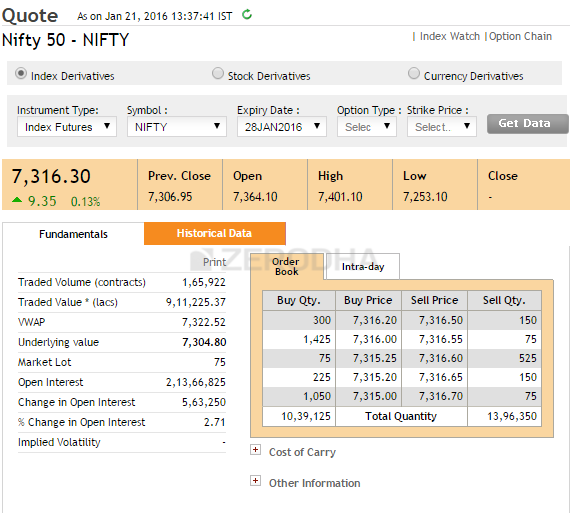On 21st Jan, Nifty spot was at 7304, and the Nifty Futures was trading at 7316.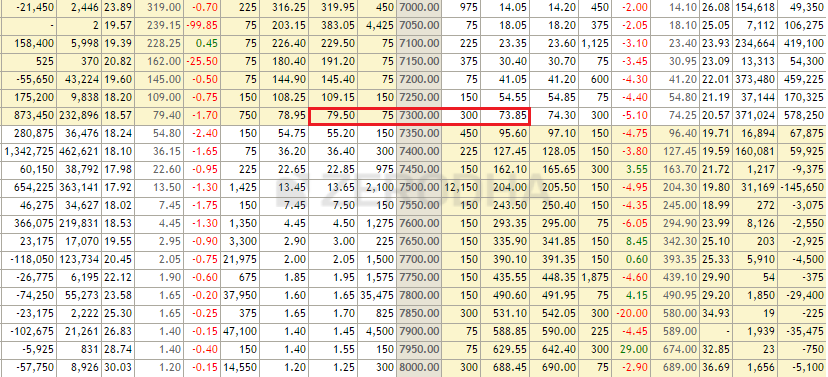The 7300 CE and PE (ATM options) were trading at 79.5 and 73.85 respectively. Do note, all the contracts belong to the January 2016 series.

Going by the arbitrage equation stated above, if one were to execute the trade, the positions would be –

1. Long 7300 CE @ 79.5
2. Short 7300 PE @ 73.85
3. Short Nifty futures @ 7316

Do note, the first two positions together form a long synthetic long. Now as per the arbitrage equation, upon expiry the positions should result in a zero P&L.  Let’s evaluate if this holds true.

Scenario 1 – Expiry at 7200

• The 7300 CE would expire worthless, hence we lose the premium paid i.e 79.5
• The 7300 PE would have an intrinsic value of 100, but since we are short at 73.85, the net payoff would be 73.85 – 100 = -26.15
• We are short on futures at 7316, which would result in a profit of 116 points (7316 – 7200)
• Net payoff would be -79.5 – 26.15 + 116 = +10.35

Clearly, instead of a 0 payoff, we are experiencing a positive non zero P&L.

Scenario 2 – Expiry at 7300

• The 7300 CE would expire worthless, hence we lose the premium paid i.e 79.5
• The 7300 PE would expire worthless, hence we get to retain 73.85
• We are short on futures at 7316, which would result in a profit of 16 points (7316 – 7300)
• Net payoff would be -79.5 +73.85+16 = +10.35

Scenario 3 – Expiry at 7400

• The 7300 CE would have an intrinsic value of 100, and therefore the payoff would be 100 – 79.5 = 20.5
• The 7300 PE would expire worthless, hence we get to retain 73.85
• We are short on futures at 7316, which would result in loss of 84 points (7316 – 7400)
• Net payoff would be 20.5 + 73.85 – 84 = +10.35

You could test this across any expiry value (in other words the markets can move in any direction) but you are likely to pocket 10.35 points, upon expiry. I’d like to stress this again; this arbitrage lets you make 10.35, upon expiry.

Here is the payoff structure at different expiry values –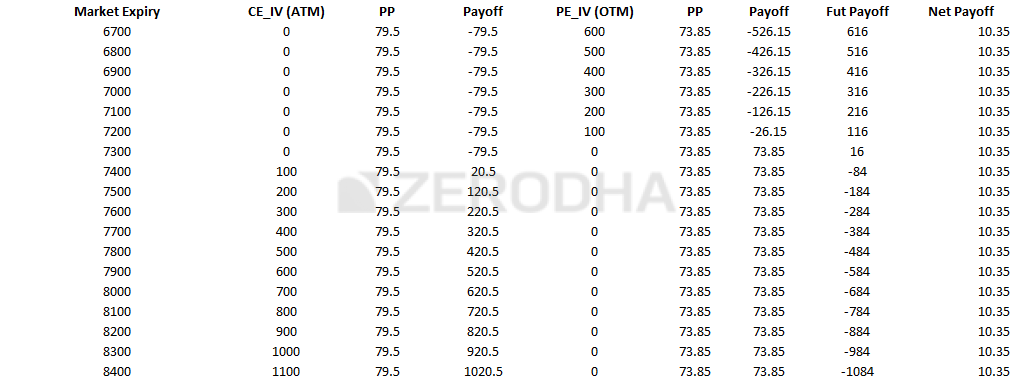Interesting isn’t it? But what’s the catch you may ask?

Transaction charges!

One has to account for the cost of execution of this trade and figure out if it still makes sense to take up the trade. Consider this –

• Brokerage – if you are trading with a traditional broker, then you will be charged on a percentage basis which will eat away your profits. So on one hand you make 10 points, but you may end up paying 8 – 10 points as brokerage. However if you were to do this trade with a discount broker like Zerodha, your breakeven on this trade would be around 4-5 points. This should give you more reason to open your account with Zerodha ☺
• STT – Do remember the P&L is realised upon expiry; hence you would have to carry forward your positions to expiry. If you are long on an ITM option (which you will be) then upon expiry you will have to pay a hefty STT, which will further eat away your profits. Please do read this to know more.
• Other applicable taxes – Besides you also have to account for service tax, stamp duty etc

So considering these costs, the efforts to carry an arbitrage trade for 10 points may not make sense. But it certainly would, if the payoff was something better, maybe like 15 or 20 points. With 15 or 20 points you can even maneuver the STT trap by squaring off the positions just before expiry – although it will shave off a few points.

### Key takeaways from this chapter

1. You can use options to replicate futures payoff
2. A synthetic long replicates the long futures payoff
3. Simultaneously buying ATM call and selling ATM Put creates a synthetic long
4. The breakeven point for the synthetic long is the ATM strike + net premium paid
5. An arbitrage opportunity is created when Synthetic long + short futures yields a positive non zero P&L upon expiry
6. Execute the arbitrage trade only if the P&L upon expiry makes sense after accounting for expenses.

1.Mahesh says:

Sir
it look very nice stratergy . but can you explain when is the right condition to enter the trade… what are the parameter i need to consider before entering this trade???

•Karthik Rangappa says:

Mahesh, you spot it ..you execute it!. There is nothing like an ideal condition for this strategy.

•Mahesh says:

thank you sir 🙂

•Karthik Rangappa says:

Welcome!

•S.K.Ahuja says:

your assumption that at 7389 nifty future and at the value of 7400PE @ Rs.80, the 7400CE can not be 127 Rs. It will be practically 69 Rs. You are calculating profit/loss at various levels of nifty expiry. In scenario one you are calculating 227 as loss. whereas in case of future the loss would be 189. In scenario 2 you are calculating 27 as loss. whereas in case of future the profit should be of Rs. 11. In scenario 3 you are calculating 0 as profit/loss. whereas in case of future the profit would be 38 . In scenario 4 you are calculating 173 as profit whereas in case of future the profit would be 211.
So in case of synthetic future strategy the P/L is always the same wherever the nifty expires. I would like to take note of the same and revert back if I am mistaken. Thanks.

•Karthik Rangappa says:

Why do you think the CE would not be 127 and why 69 specifically? Do remember that the payoff structure of synthetic long is similar to futurs @ it’s breakeven point. I would suggest you download the excel and work around with the numbers to get more clarity.

•S.K.AHUJA says:

Very poor Mr. KARTHIK RANGAPPA, Shame on the Zerodha Varsity. Can you show me any trading screen any time in the history of stock market or in future, when NIFTY FUTURES trading AT 7389, the rate of the 7400CE to be 127 and 7400PE to be 69. YOU FORGOT THE FORMULA THAT PUT PREMIUM OF ANY STRIKE + NIFTY FUTURES MUST BE EQUAL TO THE STRIKE PRICE +CALL PREMIUM. Wherever any mismatch is there the arbitration opportunity arises and immediately encashed by some trader.

•Karthik Rangappa says:

I’d suggest you download the excel once and look into the values once before concluding things. Thanks.

2.Avinash says:

with 0% risk can we earn profit in option?

•Karthik Rangappa says:

No risk, no return ! However you can minimize risk by using strategies such as Synthetic Long

3.Khyati Verdhan says:

Hi kartik
If nifty call option has premium rs.1 then how much money required to short one lot

•Karthik Rangappa says:

Margins for writing options is usually same as that of the margins required for futures. The premium value is not really considered.

4.k l agarwal client id.. RK0987 says:

what is fun for a gain of 10 to 20 rupees by investing margin of 2 lot ie 1 for future sale and 1 for put sell.appx about 90000 to 1 lacs.

•Karthik Rangappa says:

Well, a lot of professional traders like to take up trades where there is complete visibility on the downside risk even though the reward is limited.

5.rohan says:

when option strategy module will be available in pdf format like other modules are available?
how many more chapters are left?

•Karthik Rangappa says:

Rohan, the module is work in progress. I suppose another 8 chapters to go. Once this is done we will have the PDFs ready.

•Akshaya says: